Mobile QR Code1. (Department of Electrical and Computer Engineering, Seoul National University, Seoul 08826, Korea)
2. (Department of Electronic Engineering, Inha University, Incheon 22212, Korea)

Resistive random access memory, passive crossbar array, current-voltage nonlinearity

## I. INTRODUCTION

With the development of information and communication technology such as artificial intelligence, internet of things, and autonomous driving, data usage has been rapidly increasing, and faster computing systems have been required. The current computing system is configured based on a memory hierarchy to allow a central processing unit (CPU) to access lower-level memories. However, in addition to the memory wall issue which means that the gap between CPU speed and memory speed increases, limitations due to scaling of current memory technologies have been discussed . Although dynamic random access memory (DRAM), which is used for the main memory, exhibits a high speed of several tens of ns, periodic refreshing is required to prevent data loss. In addition, as DRAM cells are scaled-down, the height of the capacitor gradually increases to secure the minimum capacitance, which is also a challenging issue. Furthermore, NAND flash, which is a non-volatile memory for data storage, is also facing the limitation of scaling down. Charge-trapping flash memory enhanced stable data storage ability compared to floating-gate flash, and the high-density has been realized by stacking; however, due to the limitations of the etch technology, the number of layers cannot be infinitely increased, and the operation speed is also several hundreds of ${\mu}$s, which is a lot less than that of DRAM. To innovate the current memory hierarchy, next-generation memories have been actively investigated. The next-generation memories aim to have both the high speed of DRAM or static RAM and the non-volatility of flash memory, and include resistive (RRAM) [2-7], phase-change RAM [8-10], spin-transfer torque magnetic RAM [11-14], and ferroelectric field-effect transistor [15-17].

In particular, RRAM is a prospective candidate for next-generation memory with a simple metal-insulator-metal (MIM) structure. As the insulator material, single-layers such as HfO$_{2}$ [18,19], Al$_{2}$O$_{3}$ [20,21], TiO$_{x}$ [22,23], or bi-layers such as Al$_{2}$O$_{3}$/TiO$_{x}$ [24-28], HfO$_{2}$/TiO$_{x}$ [29,30] and HfO$_{2}$/TaO$_{x}$ [31-33] are commonly used. Depending on the formation or rupture of the conductive filament in the insulator layer, the resistance state changes to a low-resistance state (LRS) or a high resistance state (HRS). RRAM has advantages such as fast read/write speed, scalability of 4F$^{2}$, ease of 3D integration, and flexibility in material selection. However, due to a sneak path problem, it is necessary to co-integrate an additional selecting element such as a transistor or a selector for accurate cell access . The integration of a transistor and an RRAM, so-called a 1T1R structure, has a cell area of 6F$^{2}$, and its 3D integration is not an easy task. In addition, for a 1S1R structure in which an MIM selector is directly stacked on an RRAM device, it is also challenging to improve the reliability of the selector itself and to optimize operating conditions compatible with an RRAM device. Therefore, it would be ideal to configure a high-density passive array by allowing the RRAM device to have a selecting effect on its own without an external device.

Therefore, in this study, an Al$_{2}$O$_{3}$/TiO$_{x}$ bilayer-based RRAM device is fabricated and a method to enhance the nonlinear effect without the help of an external device is proposed. We adjust TiO$_{x}$ deposition conditions to utilize the fact that the electrical conductivity of TiO$_{x}$ dynamically changes depending on the oxygen concentration. This study aims to quantitatively analyze the atomic components of the TiO$_{x}$ layer and to investigate the corresponding effect on the nonlinearity of the current-voltage (I-V) properties. Also, by constructing a passive crossbar array based on the fitting parameters, we verify the effect of enhanced I-V nonlinearity on a read margin through SPICE simulation.

## II. DEVICE FABRICATION

Al$_{2}$O$_{3}$/TiO$_{x}$-based RRAM devices were fabricated with an MIM structure as shown in Fig. 1(a). The insulator layer is composed of double layers of Al$_{2}$O$_{3}$ and TiO$_{x}$, and a Ti/Pt stack was applied to both a bottom electrode (BE) and a top electrode (TE). The detailed fabrication process is as follows: First, BE was formed with 2.5 ${\mu}$m width on a SiO$_{2}$/Si substrate after initial wafer cleaning. After photolithography with negative photoresist (PR), 10 nm of adhesion layer Ti and 37 nm of Pt were deposited by e-beam evaporation. Afterward, the lift-off process was performed by removing PR with acetone solution. Next, Al$_{2}$O$_{3}$/TiO$_{x}$ oxide layers were deposited. Al$_{2}$O$_{3}$ was deposited with a thickness of 1.8 nm for 20 cycles by atomic layer deposition (ALD), using trimethylaluminum (Al(CH$_{3}$)$_{3}$, TMA) as a precursor and ozone (O$_{3}$) as a reactant, and the deposition temperature was 450$^{\circ}$C. TiO$_{x}$ was deposited with a thickness of 27~nm by direct current reactive sputtering. Nonstoichiometric TiO$_{x}$ is a material whose electrical properties such as resistivity change from metallic to semiconducting depending on oxygen concentration . Therefore, in this experiment, three samples with different oxygen concentrations of TiO$_{x}$ were fabricated by controlling the O$_{2}$ gas flow rate. They are named S1, S2, and S3, and discussed in detail later. Thereafter, the Ti/Pt TE stack was deposited with 2.5 ${\mu}$m width using the same process with BE with a thickness of 6~nm/45~nm. TE was patterned to cross vertically with BE, and thus crossbar-shaped cells were fabricated. Finally, the oxide layers were dry-etched by CHF$_{3}$ gas for electrical contact to electrodes. Electrical measurements of the fabricated RRAM devices were performed using the Keysight B1500A instrument. The voltage was swept in the positive or negative direction, and I-V characteristics were analyzed.

Fig. 1(b) shows a cross-sectional transmission electron microscopy image of the fabricated RRAM. It is confirmed that the Al$_{2}$O$_{3}$ and TiO$_{x}$ layers were deposited between TE and BE. The thickness of ALD-deposited Al$_{2}$O$_{3}$ was fixed as a control condition, and X-ray photoelectron spectroscopy (XPS) analysis was performed to confirm the change in TiO$_{x}$ composition according to the O$_{2}$ flow rate. For the XPS measurement, monochromatic Al K-Alpha X-ray source (1486.7 eV) was used. Fig. 2 shows XPS results for TiO$_{x}$ layers of three devices, S1, S2, and S3. The binding energy range for Ti atoms (450-470 eV) was mainly displayed , and four main peaks (Ti$^{0}$, Ti$^{2+}$, Ti$^{3+}$, and Ti$^{4+}$) according to the oxidation number were specified by vertical dotted lines. As a result, it was confirmed that the Ti peak compositions of the three samples were distinguished. As the O$_{2}$ flow rate increases, the intensities of Ti$^{0}$ and Ti$^{2+}$ decrease while those of Ti$^{3+}$ and Ti$^{4+}$ increase. This result implies that as the O$_{2}$ flow rate increases, the metallic component of TiO$_{x}$ decreases and the semiconducting component increases. As a result of investigating the atomic ratio with XPS, the chemical composition of TiO$_{x}$ for S1, S2, and S3 were x = 1.31, 1.52, and 1.74, respectively, confirming that oxygen concentration increased by the increase in O$_{2}$ flow rate. Note that we also tested the additional samples with Ti (corresponding to x = 0) and TiO$_{\mathrm{1.85}}$ as buffer layers. For the device with Ti, it was confirmed that the pristine state was leaky due to the oxygen scavenging effect of Ti. In addition, for the TiO$_{\mathrm{1.85}}$ sample, abnormal resistive switching was observed after the forming process. Therefore, we concluded that the samples with TiO$_{x}$ of 1.31 ${\leq}$ x ${\leq}$ 1.74 can exhibit the resistive switching properties, and compared their nonlinearities.

##### Fig. 1. (a) Schematic view and (b) cross-sectional transmission electron microscopic images of the fabricated RRAM.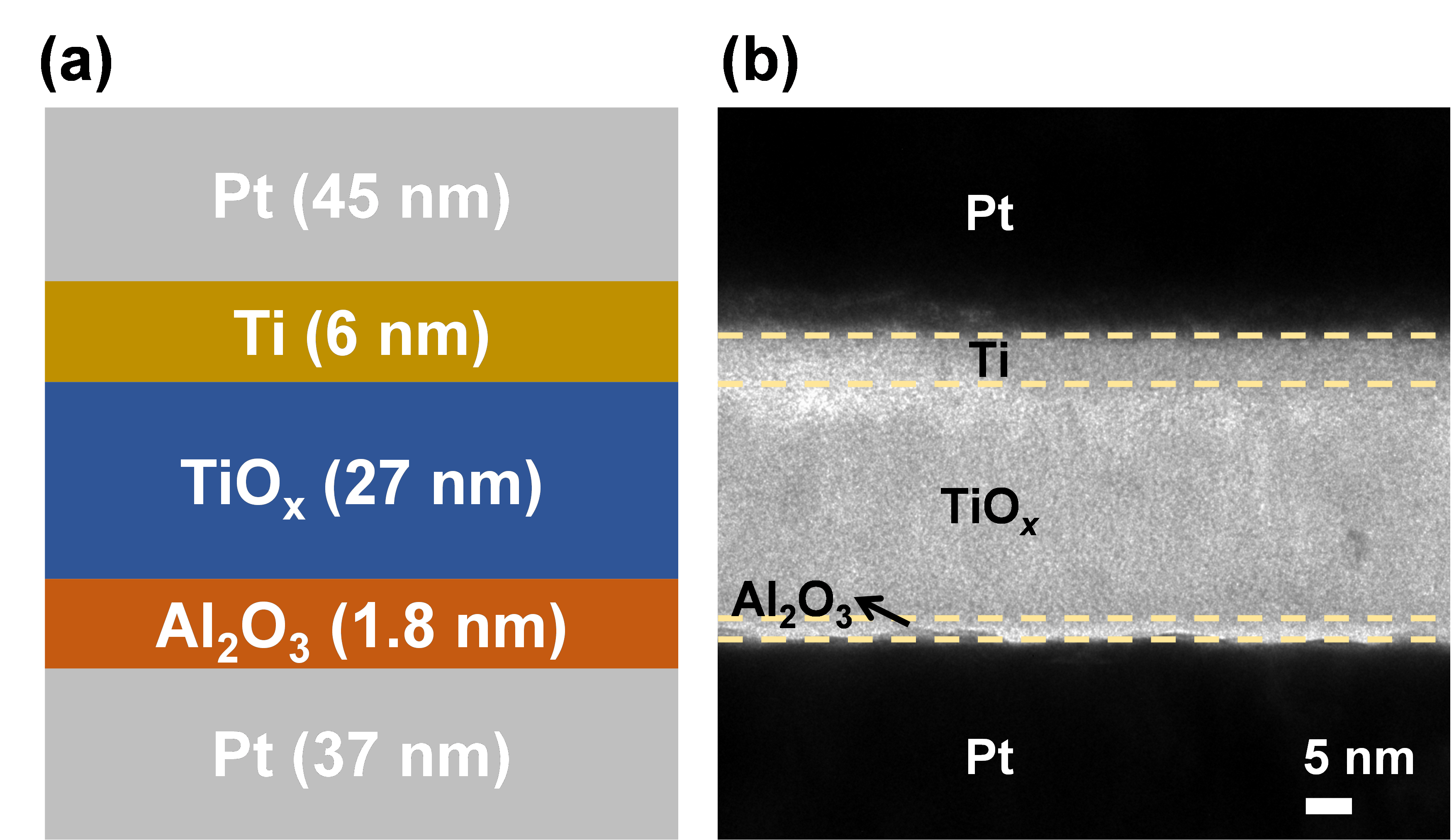##### Fig. 2. X-ray photoelectron spectroscopy (XPS) data for three devices with different oxygen concentrations of TiO$_{x}$: TiO$_{\mathrm{1.31}}$, TiO$_{\mathrm{1.52}}$, and TiO$_{\mathrm{1.74}}$.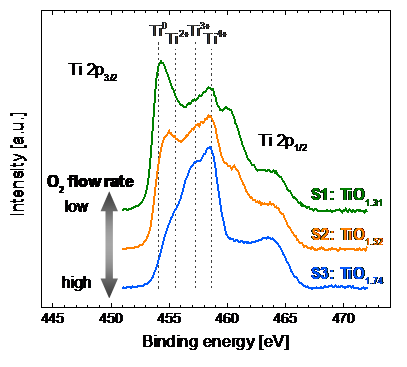## III. NONLINEARITY MEASUREMENT

Fig. 3(a)-(c) show typical I-V characteristics of S1, S2, and S3, respectively. In each figure, black, cyan, and blue lines correspond to forming, reset, and set operations, respectively. All samples were electroformed through a voltage sweep up to 2.5 V at a compliance current (I$_{\mathrm{c}}$) of 200 ${\mu}$A and switched from the initial state to LRS. I$_{\mathrm{c}}$ was set appropriately to prevent device destruction during the abrupt forming operations. Afterward, the devices were reset with a negative voltage sweep to -1.3 V, resulting in the resistive switching from LRS to HRS. For set operations, positive voltage sweeps were applied with I$_{\mathrm{c}}$ of 1 mA, and devices were switched from HRS to LRS. All samples show bipolar resistive switching characteristics, and it is confirmed that I-V nonlinearity becomes larger as the oxygen concentration of TiO$_{x}$ increases. Note that the displayed data were measured after two weeks after the fabrication, and there was no significant difference from the measured results about two years after fabrication. This result suggests that the oxygen composition of the TiO$_{x}$ layer is in a chemical equilibrium, and the stoichiometry is not affected by oxygen in the air. The semi-log I-V curves of S1, S2, and S3 are also displayed in Fig. 4 to specify rectification properties. Fig. 5 shows the forming voltage distributions of S1, S2, and S3, and the average values are specified by the black dotted line. The average forming voltages of S1, S2, and S3 were 1.980 V, 2.046~V, and 2.089 V, respectively. This is because the voltage across TiO$_{x}$ increases, as the resistivity of TiO$_{x}$ increases. As suggested in the previous work, the conductive filament is formed inside the Al$_{2}$O$_{3}$ layer, and TiO$_{x}$ acts as an oxygen reservoir . When the forming voltage is applied, the voltage is divided between the Al$_{2}$O$_{3}$ and TiO$_{x}$ layers. Thus, it can be inferred that the forming voltage is increased because more voltage is divided to TiO$_{x}$ with higher oxygen concentration.

To compare the nonlinearity, the LRS I-V characteristics of S1, S2, and S3 were normalized and fitted by the empirical model of the device . Fig. 6 shows normalized curves for three devices, and fitted lines with the following equation to quantify nonlinearity: $I=\alpha \times \sinh \left(\beta \times V\right),\,\,\left(\alpha >0,\beta >0\right),$where ${\alpha}$ is a scaling factor and ${\beta}$ determines I-V nonlinearity; the more linear the curve, the smaller ${\beta}$. As shown in Fig. 6, it is confirmed that ${\beta}$ values are 1.410, 2.375, and 2.875 in the order of S1, S2, and S3. This result suggests the electrical properties of TiO$_{x}$, which change from metallic to semiconducting conduction. As discussed earlier, the semiconducting properties of the TiO$_{x}$ layer become dominant as the oxygen concentration increases. In other words, Poole-Frenkel conduction becomes more dominant than Ohmic conduction, which can increase the nonlinearity [38,39]. For comparison of nonlinearity, an almost linear curve (${\beta}$ = 0.01) is also inserted in Fig. 6 as a black dotted line.

##### Fig. 3. Typical I-V characteristics for (a) S1; (b) S2; (c) S3. For each graph, forming, reset, and set switching curves are shown.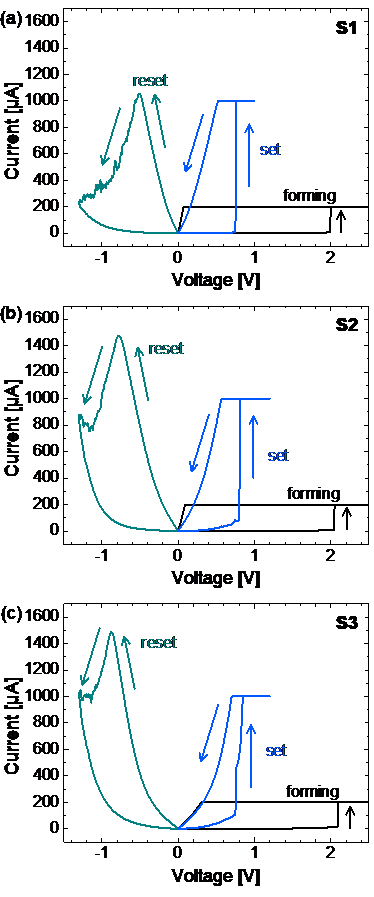##### Fig. 4. Semi-log I-V switching characteristics for S1, S2, and S3.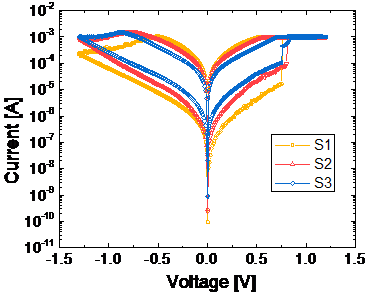##### Fig. 5. Forming voltage distributions of S1, S2, and S3.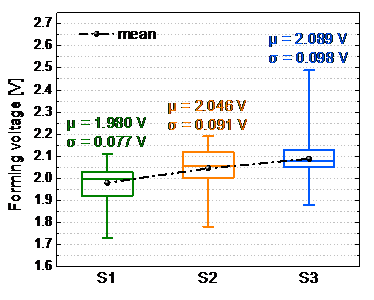##### Fig. 6. I-V nonlinearity fitting results for S1, S2, and S3. Normalized current and voltage for LRS were fitted using a hyperbolic sine function.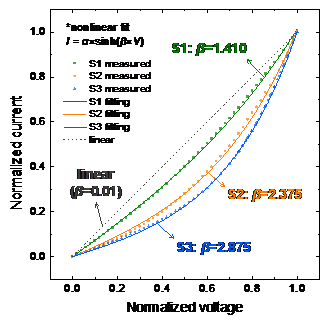To simulate the effect of nonlinearity on read margin, a passive crossbar array was constructed as shown in Fig. 7. To analyze the minimum read margin in the worst case, the cell furthest from the voltage source is selected (marked in blue), read voltage, V$_{\mathrm{read}}$, of 0.5 V is applied to the selected TE, and 0 V is applied to the selected BE. The rest unselected cells were displayed in navy and set to LRS, and a grounding read scheme was adopted to unselected TEs and BEs to improve read margin . For realistic simulation, the cell-to-cell line resistance, R$_{\mathrm{line}}$, was assumed to be 2 ${\Omega}$, which is a reasonable value because it can be realized with a 100-nm-thick Pt electrode. After configuring the crossbar array circuit, the read margin was defined as follows: $\frac{\left(I_{\mathrm{LRS}}-I_{\mathrm{HRS}}\right)}{I_{\mathrm{LRS}}}\times 100\left[\mathrm{\% }\right],$ where I$_{\mathrm{LRS}}$ and I$_{\mathrm{HRS}}$ are the total read current in the selected BE when the selected cell is LRS and HRS, respectively. Therefore, I$_{\mathrm{LRS}}$ and I$_{\mathrm{HRS}}$ include the current of the selected cell and leakage current from half-selected and unselected cells.

Fig. 8 shows the simulation results of the read margin depending on the array size, N. Because of the line resistance, the read margin decreases for all cases as the array size increases. It can be seen that as the nonlinearity increases, it is more tolerant to the reduction of the read margin. When N = 2$^{3}$ ${\times}$ 2$^{3}$, since the array size is relatively small, high read margins are obtained for all samples. However, as array size increases exponentially, the read margin decreases sharply. For example, for N = 2$^{7}$ ${\times}$ 2$^{7}$, when ${\beta}$ = 0.01 (linear) and ${\beta}$ = 1.410 (S1), the read margin is 2.32% and 5.09%, respectively. On the other hand, for ${\beta}$ = 2.375 (S2) and ${\beta}$ = 2.875 (S3), the read margin is 14.02% and 22.97%, respectively, achieving more than the minimum requirement of 10% . In conclusion, by increasing TiO$_{x}$, nonlinearity in current conduction can be increased and a high read margin for a large-scale memory array could be induced.

##### Fig. 7. Schematic circuit diagram of RRAM passive crossbar array for read margin simulation. It was configured considering cell-to-cell line resistance between cells, and the worst case is assumed. A grounding read scheme where unselected TEs and BEs are grounded is adopted.##### Fig. 8. SPICE simulation results of the read margin depending on array size N.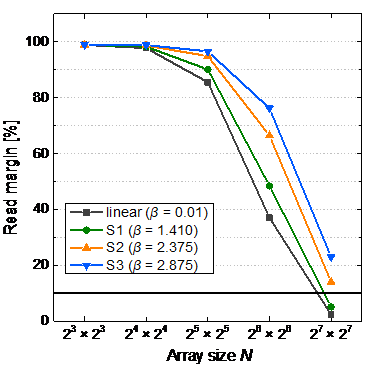## V. CONCLUSIONS

In this study, we fabricated samples S1, S2, and S3 with different resistivity of TiO$_{x}$ in Al$_{2}$O$_{3}$/TiO$_{x}$ bilayer-based RRAM devices. During the TiO$_{x}$ sputtering process, the oxygen concentration was changed by controlling the O$_{2}$ flow rate. The atomic compositions of TiO$_{x}$ for S1, S2, and S3 were found to be TiO$_{\mathrm{1.31}}$, TiO$_{\mathrm{1.52}}$, and TiO$_{\mathrm{1.74}}$, respectively, through XPS analysis. As the oxygen atomic ratio increases, Ti$^{3+}$ and Ti$^{4+}$ peaks become dominant, confirming that the semiconducting property of TiO$_{x}$ is strengthened. As a result of fitting the I-V measurement data, the nonlinearity factor ${\beta}$ values for S1, S2, and S3 were investigated as 1.410, 2.375, and 2.875, respectively. Finally, the effect of I-V nonlinearity in the passive crossbar array was confirmed through SPICE simulation. As a result, at an array size of 2$^{7}$ ${\times}$ 2$^{7}$, S1 device showed a read margin of 5.09%, which is insufficient for an accurate read operation. On the other hand, 22.97% of the read margin for S3 device was achieved despite assuming the worst case, suggesting that the high nonlinearity can effectively suppress the sneak path current caused by unselected cells. If nonlinearity is optimized by adjusting the thickness of Al$_{2}$O$_{3}$ and TiO$_{x}$ as future work, it is expected that the RRAM passive array can be utilized as a next-generation storage class memory.

## ACKNOWLEDGMENTS

This work was supported by INHA UNIVERSITY Research Grant. The authors would like to thank the BK21 FOUR Program. The EDA tool was provided by the IC Design Education Center (IDEC), Korea.

## References

1
McKee S. A., 2004., Reflections on the memory wall, in Proceedings of the 1st conference on Computing frontiers, pp. 1622
Pan F., Gao S., Chen C., Song C., Zeng F., 2014, Recent progress in resistive random access memories: Materials, switching mechanisms, performance, Mater. Sci. Eng. R-Rep., Vol. 83, pp. 1-593
Wong H.-S. P., Lee H.-Y., Yu S., Chen Y.-S., Wu Y., Chen P.-S., Lee B., Chen F. T., Tsai M.-J., 2012, Metal-oxide RRAM, Proc. IEEE, Vol. 100, No. 6, pp. 1951-19704
Jeon K., Kim J., Ryu J. J., Yoo S.-J., Song C., Yang M. K., Jeong D. S., Kim G. H., 2021, Self-rectifycing resistive memroy in passive crossbar arrays, Nat. Commun., Vol. 12, pp. 29685
Duan Y., Gao H., Guo J., Yang M., Yu Z., Shen X., Wu S., Sun Y., Ma X., Yang Y., 2021, Effect of nitrogen capture ability of quantum dots on resistive switching characteristics of AlN-based RRAM, Appl. Phys. Lett., Vol. 118, pp. 0135016
Le B. Q., Levy A., Wu T. F., Radway R. M., Hsieh E. R., Zheng X., Nelson M., Raina P., Wong H.-S. P., Wong S., Mitra S., 2021, RADAR: A fast and energy-efficient programming technique for multiple bits-per-cell RRAM arrays, IEEE Trans. Electron Devices, Vol. 68, No. 9, pp. 4397-44037
hsieh E. R., Zheng X., Le B. Q., Shih Y. C., Radway R. M., Nelson M., Mitra S., Wong S., 2021, Four-bits-per-memory one-transistor-and-eight-resistive-random-access-memory (1T8R) array, IEEE Electron Devices Lett., Vol. 42, No. 3, pp. 335-3388
Choi Y., Song I., Park M.-H., Chung H., Chang S., Cho B., Kim J., Oh Y., Kwon D., Sunwoo J., 2012, A 20nm 1.8 V 8Gb PRAM with 40MB/s program bandwidth, in 2012 IEEE International Solid-State Circuits Conference, pp. 46-489
Sarwat S. G., 2017, Materials science and engineering of phase change random access memory, Mater. Sci. Technol., Vol. 33, No. 16, pp. 1890-190610
Chen X., Ding F., Huang X., Lin X., Wang R., Chan M., Zhang L., Huang R., 2021, A robust and efficient compact mode lfor phase-change memory circuit simulations, IEEE Trans. Electron Devices, Vol. 68, No. 9, pp. 4404-441011
Khvalkovskiy A., Apalkov D., Watts S., Chepulskii R., Beach R., Ong A., Tang X., Driskill-Smith A., Butler W., Visscher P., 2013., Basic principles of STT-MRAM cell operation in memory arrays, J. Phys. D: Appl. Phys., Vol. 46, No. 7, pp. 07400112
Apalkov D., Khvalkovskiy A., Watts S., Nikitin V., Tang X., Lottis D., Moon K., Luo X., Chen E., Ong A., 2013, Spin-transfer torque magnetic random access memory (STT-MRAM), ACM J. Emerg. Technol. Comput. Syst. (JETC), Vol. 9, No. 2, pp. 1-3513
Pourmeidani H., Demara R. F., 2021, High-accuracy deep belief network: Fuzzy neural networks using MRAM-based stochastic neurons, IEEE J. Explor. Solid-State Comput. Devices Circuits, Vol. 7, No. 2, pp. 125-13114
Na T., Kang S. H., Jung S.-O., 2020, Distribution analysis and multiple-point tail fitting yield estimation method for STT-MRAM, J. Semicond. Technol. Sci., Vol. 20, No. 3, pp. 271-28015
Tan A. J., Chatterjee K., Zhou J., Kwon D., Liao Y.-H., Cheema S., Hu C., Salahuddin S., 2019, Experimental demonstration of a ferroelectric HfO$_{2}$-based content addressable memory cell, IEEE Electron Device Lett., Vol. 41, No. 2, pp. 240-24316
Bae J.-H., Kwon D., Jeon N., Cheema S., Tan A. J., Hu C., Salahuddin S., 2020, Highly scaled, high endurance, ${\Omega}$-gate, nanowire ferroelectric FET memory transistors, IEEE Electron Device Lett., Vol. 41, No. 11, pp. 1637-164017
Nguyen M.-C., Lee K., Kim S., Youn S., Hwang Y., Kim H., Choi R., Kwon D., 2022, Incremental drain-voltage-ramping training method for ferroelectric field-effect transistor synaptic devices, IEEE Electron Device Lett., Vol. 43, No. 1, pp. 17-2018
Lee D. K., Kim M.-H., Kim T.-H., Bang S., Choi Y.-J., Kim S., Cho S., Park B.-G., 2019, Synaptic behaviors of HfO$_{2}$ ReRAM by pulse frequency modulation, Solid-State Electron., Vol. 154, pp. 31-3519
Shim W., Luo Y., Seo J.-S., Yu S., 2020, Investigation of read disturb and bipolar read scheme on multilevel RRAM-based deep learning inference engine, IEEE Trans. Electron Devices, Vol. 67, No. 6, pp. 2318-232320
Sarkar B., Lee B., Misra V., 2015., Understanding the gradual reset in Pt/Al$_{2}$O$_{3}$/Ni RRAM for synaptic applications, Semicond. Sci. Technol., Vol. 30, No. 10, pp. 10501421
Mahata C., Kim M.-H., Bang S., Kim T.-H., Lee D. K., Choi Y.-J., Kim S., Park B.-G., 2019., SiO$_{2}$ layer effect on atomic layer deposition Al$_{2}$O$_{3}$-based resistive switching memory, Appl. Phys. Lett., Vol. 114, No. 18, pp. 18210222
Kim T.-H., Kim S., Park B.-G., 2021, Improved rectification characteristics by engineering energy barrier height in TiO$_{x}$-based RRAM, Microelectron. Eng., Vol. 237, pp. 11149823
Kim T.-H., Kim M.-H., Bang S., Lee D. K., Kim S., Cho S., Park B.-G., 2020, Fabrication and characterization of TiO$_{x}$ memristor for synaptic device application, IEEE Trans. Nanotechnol.24
Park J., Kim T.-H., Kim S., Lee G. H., Nili H., Kim H., 2021, Conduction mechanism effect on physical unclonable function using Al$_{2}$O$_{3}$/TiO$_{\mathrm{X}}$ memristors, Chaos Solitons Fractals, Vol. 152, pp. 11138825
Kim T.-H., Lee J., Kim S., Park J., Park B.-G., Kim H., 2021., 3-bit multilevel operation with accurate programming scheme in TiO$_{x}$/Al$_{2}$O$_{3}$ memristor crossbar array for quantized neuromorphic system, Nanotechnol., Vol. 32, No. 29, pp. 29520126
Kim T.-H., Kim S., Hong K., Park J., Hwang Y., Park B.-G., Kim H., 2021, Multilevel switching memristor by compliance current adjustment for off-chip training of neuromorphic system, Chaos Solitons Fractals, Vol. 153, pp. 11158727
Kim S., Kim T.-H., Kim H., Park B.-G., 2020., Current suppressed self-compliance characteristics of oxygen rich TiO$_{y}$ inserted Al$_{2}$O$_{3}$/TiO$_{x}$ based RRAM, Appl. Phys. Lett., Vol. 117, No. 20, pp. 20210628
Kim H., Mahmoodi M., Nili H., Strukov D. B., 2021, 4K-memristor analog-grade passive crossbar circuit, Nat. Commun., Vol. 12, pp. 519829
Zhang K., Sun K., Wang F., Han Y., Jiang Z., Zhao J., Wang B., Zhang H., Jian X., Wong H. P., 2015, Ultra-low power Ni/HfO$_{2}$/TiO$_{x}$/TiN resistive random access memory with sub-30-nA reset current, IEEE Electron Device Lett., Vol. 36, No. 10, pp. 1018-102030
Ding X., Feng Y., Huang P., Liu L., Kang J., 2019, Low-power resistive switching characteristic in HfO$_{2}$/TiO$_{x}$ Bi-layer resistive random-access memory, Nanoscale Res. Lett., Vol. 14, No. 1, pp. 1-731
Ryu H., Kim S., 2020., Self-rectifying resistive switching and short-term memory characteristics in Pt/HfO$_{2}$/TaO$_{x}$/TiN artificial synaptic device, Nanomaterials, Vol. 10, No. 11, pp. 215932
Kim S., Abbas Y., Jeon Y.-R., Sokolov A. S., Ku B., Choi C., 2018., Engineering synaptic characteristics of TaO$_{x}$/HfO$_{2}$ bi-layered resistive switching device, Nanotechnol., Vol. 29, No. 41, pp. 41520433
Ryu H., Kim S., 2021, Implementation of a reservoir computing system using the short-term effects of Pt/HfO$_{2}$/TaO$_{x}$/TiN memristors with self-rectification, Chaos Solitons Fractals, Vol. 150, pp. 11122334
Deng Y., Huang P., Chen B., Yang X., Gao B., Wang J., Zeng L., Du G., Kang J., Liu X., 2012, RRAM crossbar array with cell selection device: A device and circuit interaction study, IEEE Trans. Electron Devices, Vol. 60, No. 2, pp. 719-72635
Kim T.-H., Nili H., Kim M.-H., Min K. K., Park B.-G., Kim H., 2020., Reset-voltage-dependent precise tuning operation of TiO$_{x}$/Al$_{2}$O$_{3}$ memristive crossbar array, Appl. Phys. Lett., Vol. 117, No. 15, pp. 15210336
Chastain J., King Jr R. C., 1992, Handbook of X-ray photoelectron spectroscopy, Perkin-Elmer Corporation, Vol. 40, pp. 22137
Kim T., Kim H., Kim J., Kim J.-J., 2017, Input Voltage Mapping Optimized for Resistive Memory-Based Deep Neural Network Hardware, IEEE Electron Device Lett., Vol. 38, No. 9, pp. 1228-123138
Xu B., Sohn H. Y., Mohassab Y., Lan Y., 2016, Structures, preparation and applications of titanium suboxides, RSC Adv., Vol. 6, No. 83, pp. 79706-7972239
Chong L. H., Mallik K., de Groot C H , Kersting R., 2006, The structural and electrical properties of thermally grown TiO$_{2}$ thin films, J. Phys.-Condes. Matter, Vol. 18, No. 2, pp. 645-65740
Gao Y., Kavehei O., Al-Sarawi S. F., Ranasinghe D. C., Abbott D., 2016, Read operation performance of large selectorless cross-point array with self-rectifying memristive device, Integration, Vol. 54, pp. 56-6441
Zhou J., Kim K.-H., Lu W., 2014, Crossbar RRAM arrays: Selector device requirements during read operation, IEEE Trans. Electron Devices, Vol. 61, No. 5, pp. 1369-1376Tae-Hyeon Kim received the B.S. and Ph.D. degrees in electrical and computer engineering from Seoul National University (SNU), Seoul, Republic of Korea, in 2015 and 2022, respectively. He is currently a Postdoctoral Researcher in SNU. His current research interests include the resistive switching devices, synaptic devices, and neuromorphic systems.

Sungjoon Kim received the B.S. degree in electronics from Kyung Hee University (KHU), YongIn, Korea. And received the M.S. degree in electrical engineering from Seoul National University, Seoul, Korea. During 2010~2020, he worked for LED chip development group at Samsung Electronics Co. Ltd. He is currently working toward the Ph.D. degree in electrical engineering.

Kyungho Hong was born in Daejeon, Korea, in 1992. He received the B.S degree in electrical engineering from Seoul National University (SNU) in 2018. He is currently working toward the Ph.D. degree in electrical engineering, Seoul National University (SNU), Seoul, Korea.

Hyungjin Kim received the B.S., M.S., and Ph.D. degrees in electrical engineering from Seoul National University, Seoul, South Korea, in 2010, 2012, and 2017, respectively. He is currently an Assistant Professor with the Department of Electronic Engineering, Inha University, Incheon, Korea. His current research interests include emerging memory device, and its computing applications.

Byung-Gook Park received his B.S. and M.S. degrees in electronics engineering from Seoul National University (SNU) in 1982 and 1984, respectively, and his Ph.D. degree in electrical engineering from Stanford University in 1990. From 1990 to 1993, he worked at the AT&T Bell Laboratories, where he contributed to the development of 0.1 micron CMOS and its characterization. In 1994, he joined SNU as an assistant professor in the School of Electrical Engineering, where he is currently a professor.